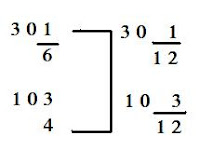### Skills Assignment 2

Wednesday, April 22, 2009
This is our Second Skills Scribe Post and it is about converting mixed fractions into improper fractions

Show 2 different ways to subtract mixed numbers:

First Question:

3 1/4 - 1 1/2 =?

You have to convert these fractions into improper fractions because if you subtract these without converting, you will end up with a negative answer.

First you have to find the LCD (lowest common denominator)

Now that we have a common denominator, we multiply the whole number with the denominator and add the left overs

The answer is still improper, so all we have to figure out is how many 4's go into 7

And now the answer is 1 3/4

Second Question:
30 1/6 - 10 3/4 =?

First you find the LCD againNow we convert 30 2/12 to 29 4/12

Now we have to subtract both fractions